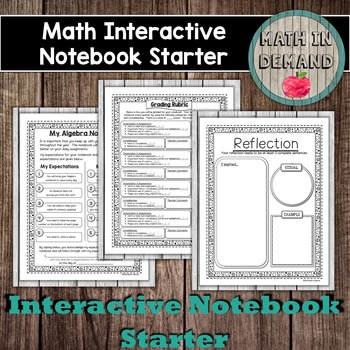# Math Interactive Notebook Starter - Algebra

Rated 5 out of 5, based on 43 reviews
43 Ratings;
6th - 12th
Subjects
Resource Type
Formats Included
• PDF
Pages
7 pages
Report this resource to TPT

### Description

This is a starter for an Algebra interactive notebook. This helps set up or start your students interactive notebooks. It will help keep your students organized and informed on how they will be graded.

Starter includes:

- Classroom Rules
- Notebook Expectations
- Reflection
- Setting Goals
- Look Back at Goals

Do you love my notebook starter and want to purchase my Algebra notebook? The Algebra notebook is an entire years worth of Algebra concepts. There are a total of 10 units that include:

Unit 1 - An introduction to Algebra concepts
Unit 2 - Equations and Inequalities
Unit 3 - Functions
Unit 4 - Sequences (Arithmetic and Geometric)
Unit 5 - Linear Equations
Unit 6 - Exponents (Powers)
Unit 7 - Systems of Equations and Inequalities
Unit 8 - Polynomials

There are two pages for each concept. The first page is used for direct instruction (notes) and the second page is for students to practice the concept. The first page includes a "I can..." statement and the second page includes an area where students can rate themselves on how well they understand the concept. This allows students to look back in their interactive notebook before a test and know which concept they need to study. There are some pages that have foldables.

I also have a Pre-Algebra interactive notebook that has the same template as my Algebra interactive notebook. There are a total of 8 units that include:

1.) Intro to Pre-Algebra
2.) Factors and Multiples
3.) Operations of Rational Numbers
4.) Rates, Ratios, & Proportions
5.) Algebraic Equations
6.) Exponents
7.) Geometry
8.) Statistics

Total Pages
7 pages